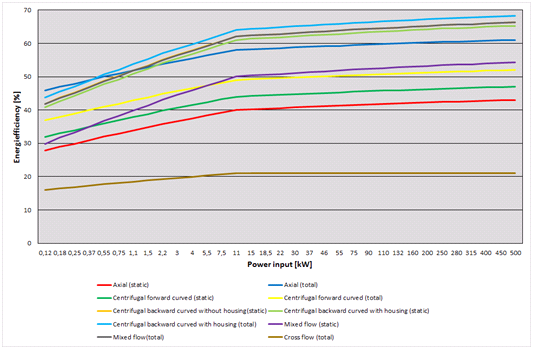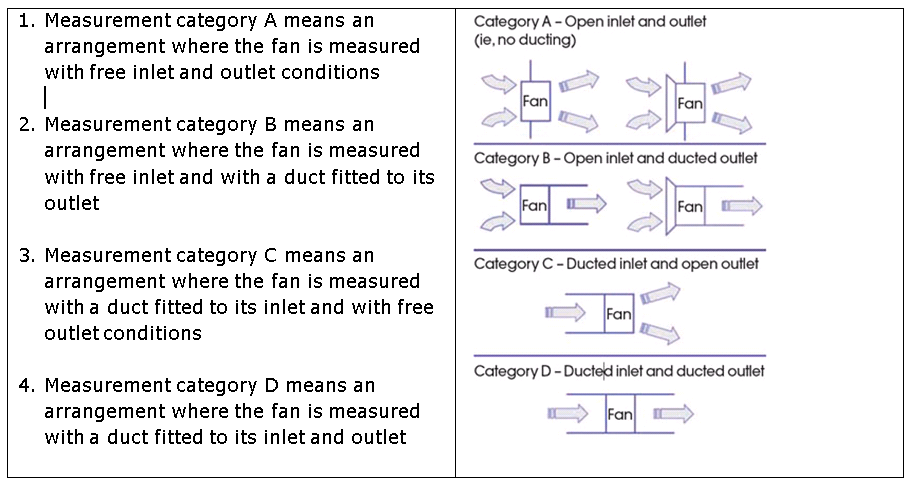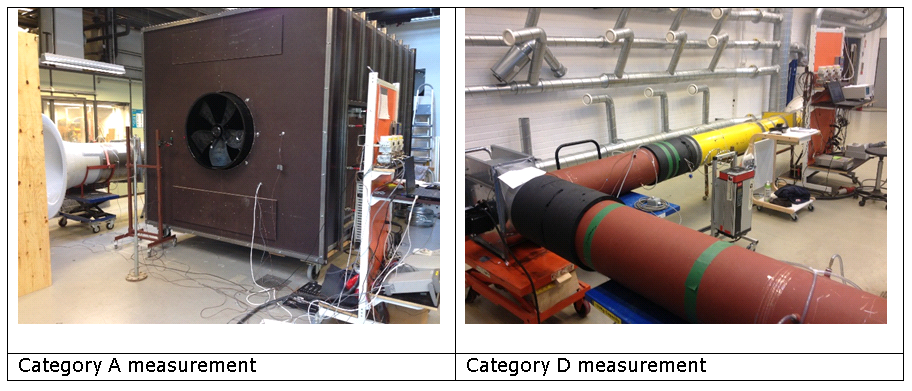INTAS
INDUSTRIAL AND TERTIARY
PRODUCT TESTING AND
APPLICATION OF STANDARDS

# Fans - efficiency measurement

​On January 1 2013, new ecodesign requirements for fans driven by electric motors came into force (Commission Regulation (EU) No 327/2011 of 30 March 2011). The requirements, which are minimum requirements, concerns fans driven by an electric motor with a power input between 125 W and 500 kW and designed at its point of optimum energy efficiency.

The requirements mean that energy efficiency is not to be measured and calculated, as the industry was accustomed to, wherein the efficiency was calculated from the static or dynamic pressure increase, the airflow and the input power of the fan. Energy efficiency is now to be measured and calculated as a total efficiency for the entire ventilation system, i.e. fan, electric motor and control.

According to the Regulation, measurements and calculations must be made using a reliable, accurate and reproducible method, which takes into account the generally recognized state-of-the-art measurement methods, and whose results are deemed to be of low uncertainty.

A measurement and calculation method that lives up to these criteria is the ISO 5801 standard for fans.

1 January 2015, the ecodesign requirements for energy efficiency were tightened up and extended to more types of fans.

The figure below shows energy requirements (target energy efficiencies) for fans applicable since 1 January 2015.Calculation method

The methodology for calculating the energy efficiency of a specific fan is based on the ratio of gas power to electrical input power to the motor, where fan gas power is the product of gas volume flow rate and pressure difference across the fan. The pressure is either the static pressure or the total pressure, which is the sum of static and dynamic pressure depending upon the measurement and efficiency category.

Measurement category means a test, measurement or usage arrangement that defines the inlet and outlet conditions of the fan under test. The categories, which are described in the ISO 5801 standard for fans, are:Final assembly

Where the fan is supplied as a ‘final assembly’, the gas power and the electric input power of the fan are measured at its optimum energy efficiency point.

Where the fan does not include a variable speed drive, the overall efficiency is calculated using the following equation:

ηe = Pu(s)/Pe

where:

• he is the overall efficiency
• Pu(s) is the fan gas power when it is operating at its optimal energy efficiency point
• Pe is the power measured at the mains input terminals to the motor of the fan when the fan is operating at its optimal energy efficiency point

where the fan includes a variable speed drive, calculate the overall efficiency using the following equation:

ηe = (Pu(s)/Pe) ∙ Cc

where:

• he is the overall efficiency
• Pu(s) is the fan gas power when it is operating at its optimal energy efficiency point
• Pe is the power measured at the mains input terminals to the variable speed drive of the fan when the fan is operating at its optimal energy efficiency point
• Cc is a part load compensation factor as follows:
• for a motor with a variable speed drive and Ped≥ 5 kW, then Cc = 1,04
• for a motor with a variable speed drive and Ped < 5 kW, then Cc = – 0,03 ln(Ped) + 1,088

Not final assembly

Where the fan is supplied as ‘not final assembly’, the fan overall efficiency is calculated at the impeller’s optimum energy efficiency point, using the following equation:

he = hr · hm · hT · Cm · Cc

where:

• he is the overall efficiency
• hr is the fan impeller efficiency according to Pu(s)/Pa

where:

• Pu(s) is fan gas power determined at the point of optimal energy efficiency for the impeller
• Pa is the fan shaft power at the point of optimal energy efficiency of the impeller
• ηm is the nominal rated motor efficiency in accordance with Regulation (EC) No 640/2009 whenever applicable
• ηT is the efficiency of the driving arrangement
• Cm is the compensation factor to account for matching of components
• Cc is the part load compensation factor

A more detailed description of this method is shown in Commission Regulation (EU) No 327/2011 and Regulation (EC) No 640/2009.

Fan gas power, Pu(s)

The fan gas power, Pu(s) (kW), is calculated according to the measurement category test method chosen by the fan supplier:

1. where the fan has been measured according to measurement category A, fan static gas power Pus is used from the equation Pus = q · psf · kps
1. where the fan has been measured according to measurement category B, fan gas power

Pu is used from the equation Pu = q · pf · kp

1. where the fan has been measured according to measurement category C, fan static gas power Pus is used from the equation Pus = q · psf · kps
1. where the fan has been measured according to measurement category D, fan gas power

Pu is used from the equation Pu = q · pf · kp

Where

• q is the airflow
• psf is the fan static pressure which means the fan total pressure pf minus the fan dynamic pressure corrected by the Mach factor.
• pf is the fan total pressure which means the difference between the stagnation pressure at the fan outlet and the stagnation pressure at the fan inlet
• The stagnation pressure means the pressure measured at a point in a flowing gas if it were brought to rest via an isentropic process
• The Mach factor means a correction factor applied to dynamic pressure at a point, defined as the stagnation pressure minus the pressure with respect to absolute zero pressure which is exerted at a point at rest relative to the gas around it and divided by the dynamic pressure
• kps and kp are compressibility factors. The value is approximately 1.

At air velocities below 40 m/s the error by calculating the difference between the static or the total pressure at the outlet and the inlet in the traditional way is less than 1 %.

Electric motors

Minimum energy efficiency requirements have been established for electric motors in Commission Regulation (EC) No 640/2009 of 22 July 2009 implementing Directive 2005/32/EC of the European Parliament and of the Council with regard to ecodesign requirements for electric motors, including electric motors equipped with variable speed drives. They also apply to those motors which are part of a motor-fan system. However, many fans covered by this Regulation are used in combination with motors not covered by Regulation (EC) No 640/2009. That is motors like permanent magnet motors (e.g. EC motors) or synchronous reluctance motors. These types of motors are specified to operate exclusively with a VSD (motors that cannot be used directly online) and are excluded from the Regulation.